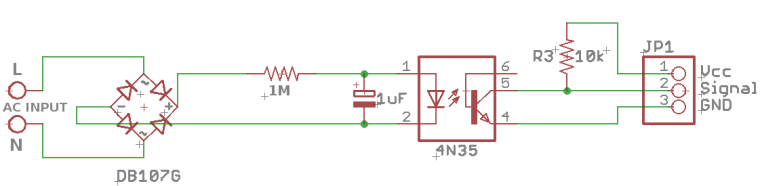Detect Generator running

• Hi all,
I'm using the circuit below to detect when my generator is running, I can see PIN 3 goes low, the signal PIN2 from the circuit is connected to D3 on the Pro-MiniI'm using the motion sketch to test with and the interrupt is seen when AC voltage is activated, but while the AC voltage is on, I get switching between high and low, how can I change the program to detect when the AC voltage is present, send a message and go to sleep. When the pin changes state again, IE AC voltage is not present anymore, the interrupt is detected and another message is send and go back to sleep until the next interrupt

Below is the code I'm using

#define MY_DEBUG

#define MY_RFM69_FREQUENCY RF69_433MHZ  // Define for frequency setting. Needed if you're radio module isn't 868Mhz (868Mhz is default in lib)
#define MY_IS_RFM69HW  // Mandatory if you radio module is the high power version (RFM69HW and RFM69HCW), Comment it if it's not the case
//#define MY_RFM69_NETWORKID 101  // Default is 100 in lib. Uncomment it and set your preferred network id if needed

#include <MySensors.h>

unsigned long SLEEP_TIME = 120000; // Sleep time between reports (in milliseconds)
#define DIGITAL_INPUT_SENSOR 3   // The digital input you attached your motion sensor.  (Only 2 and 3 generates interrupt!)
#define CHILD_ID 15   // Id of the sensor child

// Initialize motion message
MyMessage msg(CHILD_ID, V_TRIPPED);

void setup()
{
pinMode(DIGITAL_INPUT_SENSOR, INPUT);      // sets the motion sensor digital pin as input
}

void presentation()
{
// Send the sketch version information to the gateway and Controller
sendSketchInfo("Motion Sensor", "8.0");

// Register all sensors to gw (they will be created as child devices)
present(CHILD_ID, S_MOTION);
}

void loop()
{
bool tripped = digitalRead(DIGITAL_INPUT_SENSOR) == HIGH;

Serial.println(tripped);
//send(msg.set(tripped?"1":"0"));  // Send tripped value to gw

// Sleep until interrupt comes in on motion sensor. Send update every two minute.
sleep(digitalPinToInterrupt(DIGITAL_INPUT_SENSOR), CHANGE, SLEEP_TIME);
}

The Serial output is below showing the switching between high and low

0 MCO:BGN:INIT NODE,CP=RRNNA--,VER=2.1.1
4 TSM:INIT
4 TSF:WUR:MS=0
8 TSM:INIT:TSP OK
10 TSF:SID:OK,ID=10
12 TSM:FPAR
143 TSF:MSG:SEND,10-10-255-255,s=255,c=3,t=7,pt=0,l=0,sg=0,ft=0,st=OK:
288 TSF:MSG:FPAR OK,ID=0,D=1
2150 TSM:FPAR:OK
2150 TSM:ID
2152 TSM:ID:OK
2154 TSM:UPL
2162 TSF:MSG:SEND,10-10-0-0,s=255,c=3,t=24,pt=1,l=1,sg=0,ft=0,st=OK:1
2220 TSF:MSG:PONG RECV,HP=1
2224 TSM:UPL:OK
2236 TSF:MSG:SEND,10-10-0-0,s=255,c=3,t=15,pt=6,l=2,sg=0,ft=0,st=OK:0100
2304 TSF:MSG:SEND,10-10-0-0,s=255,c=0,t=17,pt=0,l=5,sg=0,ft=0,st=OK:2.1.1
2318 TSF:MSG:SEND,10-10-0-0,s=255,c=3,t=6,pt=1,l=1,sg=0,ft=0,st=OK:0
4335 TSF:MSG:SEND,10-10-0-0,s=255,c=3,t=11,pt=0,l=13,sg=0,ft=0,st=OK:Motion Sensor
4354 TSF:MSG:SEND,10-10-0-0,s=255,c=3,t=12,pt=0,l=3,sg=0,ft=0,st=OK:8.0
4368 TSF:MSG:SEND,10-10-0-0,s=15,c=0,t=1,pt=0,l=0,sg=0,ft=0,st=OK:
4376 MCO:REG:REQ
4384 TSF:MSG:SEND,10-10-0-0,s=255,c=3,t=26,pt=1,l=1,sg=0,ft=0,st=OK:2
4442 MCO:PIM:NODE REG=1
4446 MCO:BGN:STP
4448 MCO:BGN:INIT OK,TSP=1
1
4450 MCO:SLP:MS=120000,SMS=0,I1=1,M1=1,I2=255,M2=255
4456 MCO:SLP:TPD
4458 MCO:SLP:WUP=1
1
4460 MCO:SLP:MS=120000,SMS=0,I1=1,M1=1,I2=255,M2=255
4466 MCO:SLP:TPD
4468 MCO:SLP:WUP=1
1
4470 MCO:SLP:MS=120000,SMS=0,I1=1,M1=1,I2=255,M2=255
4476 MCO:SLP:TPD
4478 MCO:SLP:WUP=1
0
4483 MCO:SLP:MS=120000,SMS=0,I1=1,M1=1,I2=255,M2=255
4487 MCO:SLP:TPD
4489 MCO:SLP:WUP=1
0
4493 MCO:SLP:MS=120000,SMS=0,I1=1,M1=1,I2=255,M2=255
4497 MCO:SLP:TPD
4499 MCO:SLP:WUP=1
1
4503 MCO:SLP:MS=120000,SMS=0,I1=1,M1=1,I2=255,M2=255
4509 MCO:SLP:TPD
4511 MCO:SLP:WUP=1
1
4513 MCO:SLP:MS=120000,SMS=0,I1=1,M1=1,I2=255,M2=255
4519 MCO:SLP:TPD
4521 MCO:SLP:WUP=1
0
4524 MCO:SLP:MS=120000,SMS=0,I1=1,M1=1,I2=255,M2=255
4530 MCO:SLP:TPD
4532 MCO:SLP:WUP=1
1

Any help or ideas on how to do this would be appreciated please

• Hello,

Why is the input switching when the generator is on ? Is it switching at 50Hz (or 60Hz) or for an other reason ?

If it is switching at 50Hz, simply require the signal to stay low for >0,02s before considering the generator is off. (As a general rule, it is a good idea to have these debounce timers.)

If it is switching for an other reason, you'll have to find why it is doing so. A multimeter will probably of great help (or just use Serial.println(), comment the sleep() function and connect your computer to the Arduino.)

• @esawyja

The rectifier is unnecessary, the optocoupler has an built-in diode. The 1 m resistor can burn. You'll have an voltage not much smaller than the input voltage at it. I would recommend to replace it with an capacitor.

• @esawyja have you tried setting the sleep time to 0 ? at the moment you are waking up the sensor every 2 minutes and it is sending data, if you set sleep time to 0 it will only wake when it detects a change in the interrupt.

unsigned long SLEEP_TIME = 0; // Sleep time between reports (in milliseconds)

• Thanks All,
I got it sort of working using the original circuit with this sketch below, using another variable with last runstate, sorry my coding is shocking.,...

#define MY_DEBUG

#define MY_RFM69_FREQUENCY RF69_433MHZ  // Define for frequency setting. Needed if you're radio module isn't 868Mhz (868Mhz is default in lib)
#define MY_IS_RFM69HW  // Mandatory if you radio module is the high power version (RFM69HW and RFM69HCW), Comment it if it's not the case
//#define MY_RFM69_NETWORKID 101  // Default is 100 in lib. Uncomment it and set your preferred network id if needed

#include <MySensors.h>

unsigned long SLEEP_TIME = 3600000; // Every hour Sleep time between reports (in milliseconds)
#define DIGITAL_INPUT_SENSOR 3   // The digital input you attached your motion sensor.  (Only 2 and 3 generates interrupt!)
#define CHILD_ID 15   // Id of the sensor child

int laststate=0;

// Initialize motion message
MyMessage msg(CHILD_ID, V_TRIPPED);

void setup()
{
pinMode(DIGITAL_INPUT_SENSOR, INPUT);      // sets the motion sensor digital pin as input
}

void presentation()
{
// Send the sketch version information to the gateway and Controller
sendSketchInfo("Motion Sensor", "8.0");

// Register all sensors to gw (they will be created as child devices)
present(CHILD_ID, S_MOTION);
}

void loop()
{
bool tripped = digitalRead(DIGITAL_INPUT_SENSOR) == LOW;
if ( tripped==1) {
laststate=1;
Serial.print("Generator State =  ");
Serial.println(tripped);
}
if (( tripped==1 ) & (laststate==1)) {
//Serial.println("Still Running");
delay(60000);
}
if (tripped==0) {
laststate=0;
Serial.println("Generator OFF");
//send(msg.set(tripped?"1":"0"));  // Send tripped value to gw

// Sleep until interrupt comes in on motion sensor. Send update every two minute.
sleep(digitalPinToInterrupt(DIGITAL_INPUT_SENSOR), HIGH, SLEEP_TIME);
}
}

5

4

4

4

3

3

14